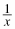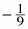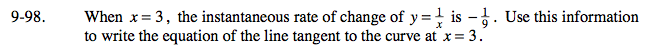### Home > PC > Chapter 9 > Lesson 9.3.1 > Problem9-98

9-98.

When x = 3, the instantaneous rate of change of y =is. Use this information to write the equation of the line tangent to the curve at x = 3. Homework Help ✎The instantaneous rate of change is the slope.

Evaluate the equation of the curve for x = 3. Now you have a point on the tangent line.